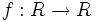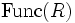# Ring of functions from a ring to itself

(diff) ← Older revision | Latest revision (diff) | Newer revision → (diff)
Let$R$ be a commutative unital ring. The ring of functions from$R$ to itself is the ring of all functions$f: R \to R$ with pointwise addition and multiplication.
This ring is sometimes denoted$\operatorname{Func}(R)$, sometimes$F(R,R)$.